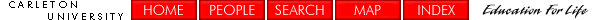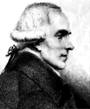Calculus and Calculus-related Pages

Pre-Calculus for Science and Engineering Students: Mathematics 69.007 Section A -- Fall, 1996
Calculus for Engineering Students: Mathematics 69.104 Section A -- Fall, 1996
Calculus for Science Students: Mathematics 69.107 Sections K & L-- Fall, 1997
Differential Equations and Infinite Series for Engineering Students: Mathematics 69.105 Section D-- Winter, 1998
Multivariable Calculus for Engineering Students : Mathematics 69.204 Section B-- Winter, 1998
Calculus for Science Students: Mathematics 69.107 Sections A,B,C, and D-- Fall, 1999
Calculus for Engineering Students: Mathematics 69.104 Sections A,B,C, and D-- Fall, 1999-2004
Calculus for Mathematics and other Honors students: Mathematics 69.102 -- Fall/Winter, 2001-2002
Calculus for Engineering Students: Mathematics 69.104 Section A -- Fall, 2001
Theory of Ordinary Differential Equations: Mathematics 3008 -- Winter, 2004
Calculus for Engineering Students: Mathematics 69.1004 Sections A, B, C -- Fall, 2005
Calculus for Science Students: Mathematics 69.1007 Section D -- Fall, 2005

Detailed Course Outline for Mathematics 69.107/69.104Sir Isaac Newton, co-discoverer of Calculus

What is Calculus? Derivatives: Definition, rules, implicit differentiation, derivatives of trigonometric functions, Newton's method, L'Hopital's rule, exponential and logarithmic functions, curve sketching, logarithmic differentiation using L'Hopital's rule, exponential growth and decay, simple differential equations, inverse trigonometric functions, word problems, definite integrals, Fundamental Theorem of Calculus, numerical integration, integration by substitution (change of variable), trigonometric integrals area between curves, integration by parts, volume by cylindrical shells, more volumes, trigonometric integrals, partial fractions, separable differential equations, more differential equations.

For a detailed weekly schedule of the course Math 69.107 for the Fall term, 1997, follow this link.
For a detailed weekly schedule of the course Math 69.104 for the Fall term, 1999, follow this link.
For a detailed weekly schedule of the course Math 69.104 for the Fall term, 2004, follow this link.
For a detailed weekly schedule of the course Math 69.107 for the Fall term, 2005, follow this link.
For a detailed weekly schedule of the course Math 69.1004 for the Fall term, 2005, follow this link.

Engineering Calculus

Differential Equations and Infinite Series for Engineering Students Mathematics 69.105 Section D
Multivariable Calculus for Engineering Students Mathematics 69.204 Section B
Mathematics 69.104 Section A (Fall, 1996)
Mathematics 69.104 Section A (Fall, 2004)Pierre Simon Marquis de Laplace, discoverer of the Laplace Transform

Pre-Calculus

Mathematics 69.007 Section A (Fall, 1996)
websurfers served since April 11, 1997